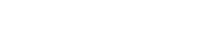# ISA Certified Control Systems Technician (CCST) program

• March 31, 2016
• CCST

Certified Control System Technicians (CCSTs) calibrate, document, troubleshoot, and repair/replace instrumentation for systems that measure and control level, temperature, pressure, flow, and other process variables.

### CCST question

A fluid is flowing through a 10-inch diameter pipe at a velocity of 6 ft/sec. When the pipe reduces to an 8-inch diameter, and all other flowing parameters remain the same, the fluid velocity becomes ________ ft/sec.

A. 2.550
B. 6.075
C. 9.375
D. 12.75

The correct answer is C, 9.375 ft/sec.

Flow in a pipe can be expressed as:
Q = A x V,

where

Q = volumetric flow rate, ft3/sec
A = pipe cross-sectional area, ft2
V = fluid velocity, ft/sec

The flow rate of the fluid through the 10-inch pipe (condition 1) is the same as the flow rate through the 8-inch pipe (condition 2), so:

Q1 = Q2

and since Q = A x V,

A1 x V1 = A2 x V2

Solving for V2:

V2 = A1 x V1 / A2 = [A1 / A2 ] x V1

Area is proportional to the square of the pipe diameter (A = Π x D2 / 4), so:

V2 = [(10 in)2 / (8 in)2] x 6 ft/sec

V2 = 9.375 ft/sec

Reference: Goettsche, L.D. (Editor), Maintenance of Instruments and Systems, Second Edition, ISA, 2005.